## Wednesday, December 10, 2008

### Irodov Problem 1.251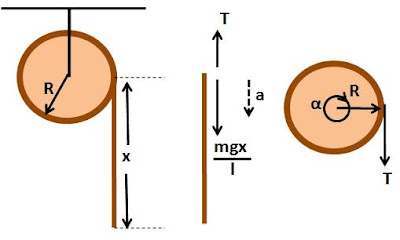At any instant if x is the length of the hanging part of the cord then the mass of the hanging part of the cord is given byand so the force of gravity acting on it is given by. This means that as more and more cord starts to hang (x increases), the force of gravity acting on the cord also increases.
There are two forces acting at the topmost point of the hanging part of the cord, i) the tension from the end point of rest of cord (non haging part) wound on the cylinder T and ii) the force of gravity of the hanging part of the cord. Suppose that the downward acceleration of the cord is given by a. Then from Newton's laws we have,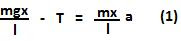Now let us consider the rotation of the cylinder. The combined moment of inertia of the cylinder + cord system about its axis of rotation is the sum of moment of intertia of two parts i) the solid cylinder of mass M and radius R given by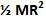and ii) the moment of interia of the cord wound on the cylinder. The mass of the wound part (non-hanging part) of the cord is given byand each point of the cord is at a distance R from the axis of rotation of the cylinder. Thus, the moment of inertia of the wound part of the cord is given by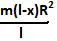. The total moment of interia of the cylinder + cord system is given by,The tension acting on the end point of the wound part of the cord will induce a torque on the cylinder + cord system and will be equal to TR. Let the angular acceleration of the cylinder + cord system be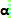. Then we have,Since there is no slipping between the cord and the surface of the cylinder we have,Now we have all the equations necessary to solve the problem. From (1),(2),(3) and (4) we have,Anonymous said...

what about torque due to mass of the cord?

Krishna Kant Chintalapudi said...

Tension T already includes the effects due to the mass of the cord.

We have divided the system into two free bodies - a) the hanging part of the cord and b) the cylinder with the unwound part of the cord.

Tension T is the only force acting on b), while T and gravity both act on a). Basically think of it as two separate system a) and b).Anonymous said...